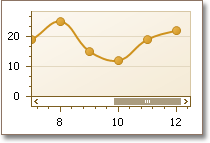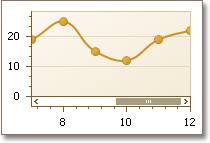21.2
21.1
20.2
20.1
19.2
19.1
18.2
18.1
17.2

# Range.AutoSideMargins Property

Specifies whether a space should be left between the outermost series point and the diagram’s edge, when it is scrolled.

Namespace: DevExpress.XtraCharts

Assembly: DevExpress.XtraCharts.v21.2.dll

## Declaration

``public bool AutoSideMargins { get; set; }``

## Property Value

Type Description
Boolean

true, if the series are plotted with indents; otherwise, false.

## Remarks

Use the AutoSideMargins property, to force an axis to automatically extend its scrolling range so that indents are preserved on both ends of the axis.

The following images demonstrate how the AutoSideMargins property works.

AutoSideMargins = true AutoSideMargins = false## Example

This example demonstrates how to use the AxisBase.VisualRange property to define the visible range of an axis, and the AxisBase.WholeRange property to define its whole range.

For more information on axis range, refer to Visual Ranges and Whole Ranges .

``````using DevExpress.XtraCharts;
using System;
using System.Windows.Forms;

namespace WindowsFormsApplication1 {
public partial class Form1 : Form {
public Form1() {
InitializeComponent();
}

private void Form1_Load(object sender, EventArgs e) {

// Create a chart control.
ChartControl chartControl1 = new ChartControl();

// Add the chart to the form.
chartControl1.Dock = DockStyle.Fill;

// Create a bar series and add points to it.
Series series1 = new Series("Series 1", ViewType.Bar);
series1.Points.Add(new SeriesPoint("A", new double[] { 26.25 }));
series1.Points.Add(new SeriesPoint("B", new double[] { 1.52 }));
series1.Points.Add(new SeriesPoint("C", new double[] { 22.21 }));
series1.Points.Add(new SeriesPoint("D", new double[] { 15.35 }));
series1.Points.Add(new SeriesPoint("E", new double[] { 4.15 }));

// Add the series to the chart.

// Cast the chart's diagram to the XYDiagram type, to access its axes.
XYDiagram diagram = (XYDiagram)chartControl1.Diagram;

// Enable the diagram's scrolling.
diagram.EnableAxisXScrolling = true;
diagram.EnableAxisYScrolling = true;

// Define the whole range for the X-axis.
diagram.AxisX.WholeRange.Auto = false;
diagram.AxisX.WholeRange.SetMinMaxValues("A", "D");

// Disable the side margins
// (this has an effect only for certain view types).
diagram.AxisX.VisualRange.AutoSideMargins = false;

// Limit the visible range for the X-axis.
diagram.AxisX.VisualRange.Auto = false;
diagram.AxisX.VisualRange.SetMinMaxValues("B", "C");

// Define the whole range for the Y-axis.
diagram.AxisY.WholeRange.Auto = false;
diagram.AxisY.WholeRange.SetMinMaxValues(1, 26);
}
}
}
``````